# 2.1: Polar Covalent Bonds - Electronegativity

$$\newcommand{\vecs}{\overset { \rightharpoonup} {\mathbf{#1}} }$$ $$\newcommand{\vecd}{\overset{-\!-\!\rightharpoonup}{\vphantom{a}\smash {#1}}}$$$$\newcommand{\id}{\mathrm{id}}$$ $$\newcommand{\Span}{\mathrm{span}}$$ $$\newcommand{\kernel}{\mathrm{null}\,}$$ $$\newcommand{\range}{\mathrm{range}\,}$$ $$\newcommand{\RealPart}{\mathrm{Re}}$$ $$\newcommand{\ImaginaryPart}{\mathrm{Im}}$$ $$\newcommand{\Argument}{\mathrm{Arg}}$$ $$\newcommand{\norm}{\| #1 \|}$$ $$\newcommand{\inner}{\langle #1, #2 \rangle}$$ $$\newcommand{\Span}{\mathrm{span}}$$ $$\newcommand{\id}{\mathrm{id}}$$ $$\newcommand{\Span}{\mathrm{span}}$$ $$\newcommand{\kernel}{\mathrm{null}\,}$$ $$\newcommand{\range}{\mathrm{range}\,}$$ $$\newcommand{\RealPart}{\mathrm{Re}}$$ $$\newcommand{\ImaginaryPart}{\mathrm{Im}}$$ $$\newcommand{\Argument}{\mathrm{Arg}}$$ $$\newcommand{\norm}{\| #1 \|}$$ $$\newcommand{\inner}{\langle #1, #2 \rangle}$$ $$\newcommand{\Span}{\mathrm{span}}$$

##### Objectives

After completing this section, you should be able to

1. describe how differences in electronegativity give rise to bond polarity.
2. arrange a given series of the elements most often encountered in organic chemistry (C, H, O, N, S, P and the halogens) in order of increasing or decreasing electronegativity, without referring to a table of electronegativities.
3. predict the partial positive and partial negative ends of a given bond formed between any two of the elements listed in Objective 2, above, without the use of a table of electronegativities or a periodic table.
4. predict the partial positive and partial negative ends of a given bond formed between any two elements not listed in Objective 2, above, using a periodic table.
##### Key Terms

Make certain that you can define, and use in context, the key terms below.

• electronegativity inductive effect
• polar covalent bond
##### Study Notes

Students often wonder why it is important to be able to tell whether a given bond is polar or not, and why they need to know which atoms carry a partial positive charge and which a partial negative charge. Consider the chloromethane (CH3Cl) molecule. The carbon atom is shown as carrying a partial positive charge. Now, recall that opposite charges attract. Thus, it seems reasonable that the slightly positive carbon atom in chloromethane should be susceptible to attack by a negatively charged species, such as the hydroxide ion, OH. This theory is borne out in practice: hydroxide ions react with chloromethane by attacking the slightly positive carbon atom in the latter. It is often possible to rationalize chemical reactions in this manner, and you will find the knowledge of bond polarity indispensible when you start to write reaction mechanisms.

Note: Because of the small difference in electronegativity between carbon and hydrogen, the C-H bond is normally assumed to be nonpolar.

### Electronegativity

Because the tendency of an element to gain or lose electrons is so important in determining its chemistry, various methods have been developed to quantitatively describe this tendency. The most important method uses a measurement called electronegativity (represented by the Greek letter chi, χ, pronounced “ky” as in “sky”), which is defined as the relative ability of an atom to attract electrons to itself in a chemical compound. Elements with high electronegativities tend to acquire electrons in chemical reactions and are found in the upper right corner of the periodic table. Elements with low electronegativities tend to lose electrons in chemical reactions and are found in the lower left corner of the periodic table.

Electronegativity of an atom is not a simple, fixed property that can be directly measured in a single experiment. In fact, an atom’s electronegativity should depend to some extent on its chemical environment because the properties of an atom are influenced by the neighboring atoms in a chemical compound. Nevertheless, when different methods for measuring the electronegativity of an atom are compared, they all tend to assign similar relative values to a given element. Figure $$\PageIndex{1}$$ shows the electronegativity values of the elements as proposed by one of the most famous chemists of the twentieth century: Linus Pauling. In this scale a value of 4.0 is arbitrarily given to the most electronegative element, fluorine, and the other electronegativities are scaled relative to this value. In general, electronegativity increases from left to right across a period in the periodic table and decreases down a group. Thus, the nonmetals, which lie in the upper right, tend to have the highest electronegativities, with fluorine the most electronegative element of all (EN = 4.0 as previously noted). It is important to notice that the elements most important to organic chemistry, carbon, nitrogen, and oxygen have some of the highest electronegativites in the periodic table (EN = 2.5, 3.0, 3.5 respectively). Metals, on the left, tend to be less electronegative elements, with cesium having the lowest (EN = 0.7). Note that noble gases are excluded from this figure because these atoms usually do not share electrons with others atoms since they have a full valence shell.

Electronegativity is defined as the ability of an atom in a particular molecule to attract electrons to itself. The larger the electronegativity value, the greater the attraction.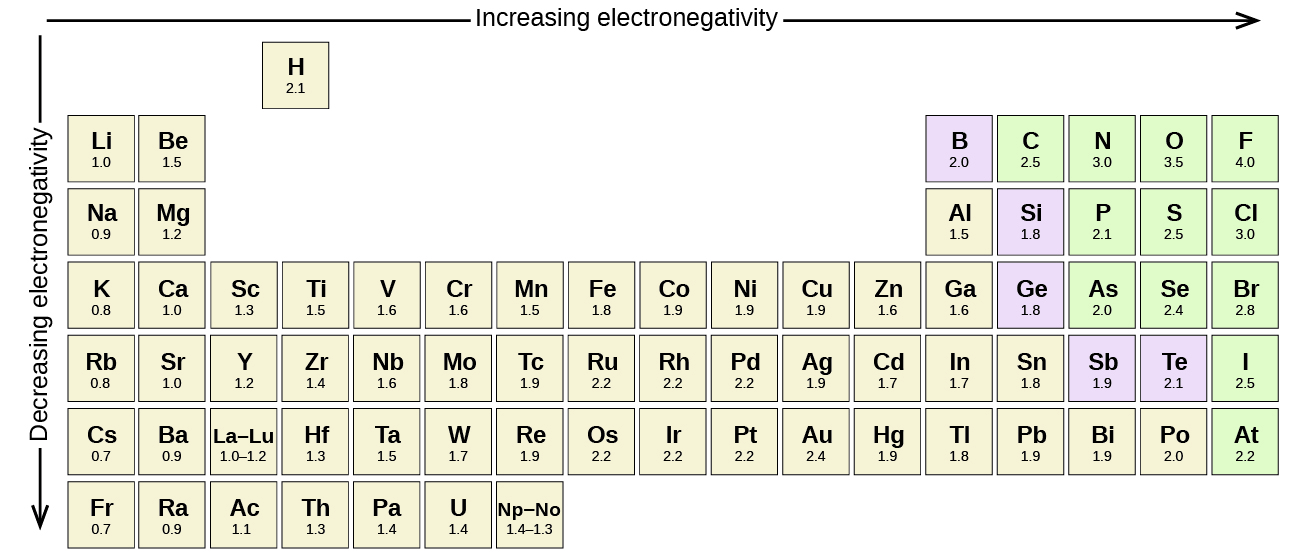Figure $$\PageIndex{1}$$: Pauling scale electronegativities of elementsFigure $$\PageIndex{2}$$: Visual representation of electronegativities.

## Electronegativity and Bond Type

The two idealized extremes of chemical bonding: (1) ionic bonding—in which one or more electrons are transferred completely from one atom to another, and the resulting ions are held together by purely electrostatic forces—and (2) covalent bonding, in which electrons are shared equally between two atoms. Most compounds, however, have polar covalent bonds, which means that electrons are shared unequally between the bonded atoms. Electronegativity determines how the shared electrons are distributed between the two atoms in a polar covalent bond. The more strongly an atom attracts the electrons in its bonds, the larger its electronegativity. Electrons in a polar covalent bond are shifted toward the more electronegative atom; thus, the more electronegative atom is the one with the partial negative charge. The greater the difference in electronegativity, the more polarized the electron distribution and the larger the partial charges of the atoms. Recall that a lowercase Greek delta ( δ ) is used to indicate that a bonded atom possesses a partial positive charge, indicated by δ+ , or a partial negative charge, indicated by δ , and a bond between two atoms that possess partial charges is a polar bond.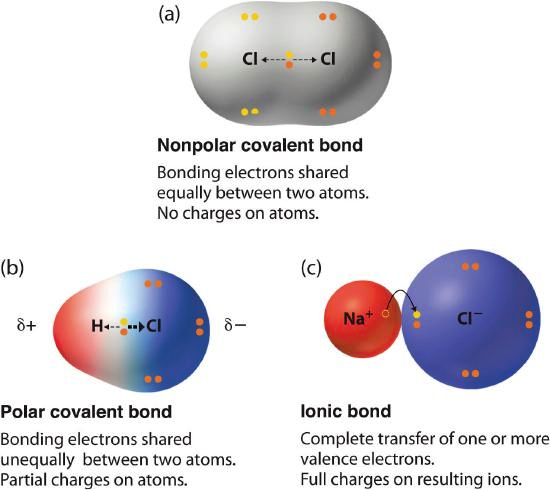Figure $$\PageIndex{3}$$: The Electron Distribution in a Nonpolar Covalent Bond, a Polar Covalent Bond, and an Ionic Bond Using Lewis Electron Structures. Electron-rich (negatively charged) regions are shown in blue; electron-poor (positively charged) regions are shown in red.

Whether a bond is ionic, nonpolar covalent, or polar covalent can be estimated by by calculating the absolute value of the difference in electronegativity (ΔEN) of two bonded atoms. When the difference is very small or zero, the bond is covalent and nonpolar. When it is large, the bond is polar covalent or ionic. The absolute values of the electronegativity differences between the atoms in the bonds H–H, H–Cl, and Na–Cl are 0 (nonpolar), 0.9 (polar covalent), and 2.1 (ionic), respectively. The degree to which electrons are shared between atoms varies from completely equal (pure covalent bonding) to not at all (ionic bonding). Figure 7.2.4 shows the relationship between electronegativity difference and bond type. This table is just a general guide, however, with many exceptions. The best guide to the covalent or ionic character of a bond is to consider the types of atoms involved and their relative positions in the periodic table. Bonds between two nonmetals are generally covalent; bonding between a metal and a nonmetal is often ionic.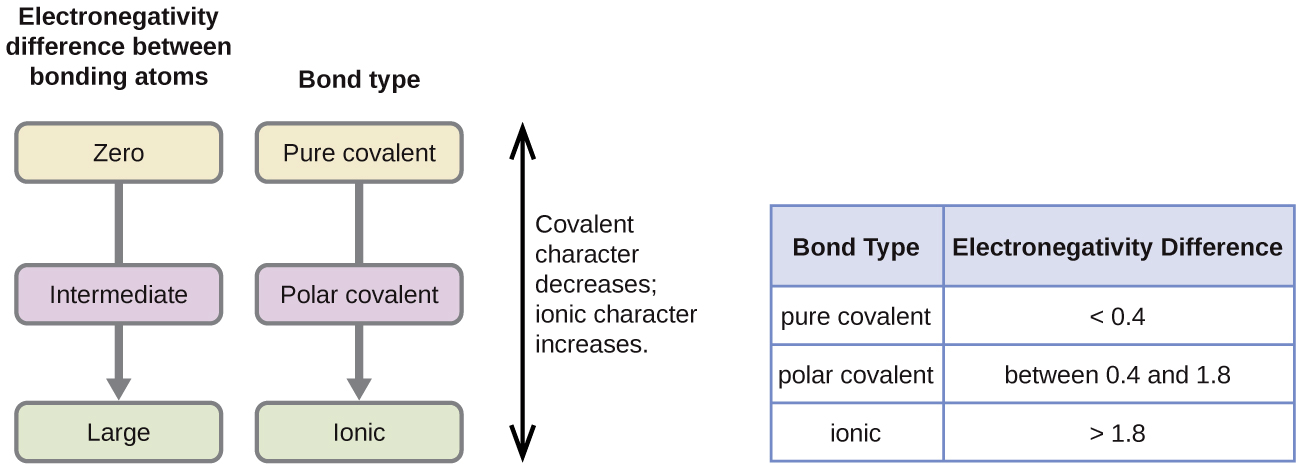Figure $$\PageIndex{4}$$: As the electronegativity difference increases between two atoms, the bond becomes more ionic.

Some compounds contain both covalent and ionic bonds. The atoms in polyatomic ions, such as OH, NO3, and NH4+, are held together by polar covalent bonds. However, these polyatomic ions form ionic compounds by combining with ions of opposite charge. For example, potassium nitrate, KNO3, contains the K+ cation and the polyatomic NO3 anion. Thus, bonding in potassium nitrate is ionic, resulting from the electrostatic attraction between the ions K+ and NO3, as well as covalent between the nitrogen and oxygen atoms in NO3.

Example $$\PageIndex{1}$$: Electronegativity and Bond Polarity

Bond polarities play an important role in determining the structure of proteins. Using the electronegativity values in Table A2, arrange the following covalent bonds—all commonly found in amino acids—in order of increasing polarity. Then designate the positive and negative atoms using the symbols δ+ and δ–:

C–H, C–N, C–O, N–H, O–H, S–H

Solution

The polarity of these bonds increases as the absolute value of the electronegativity difference increases. The atom with the δ– designation is the more electronegative of the two. Table $$\PageIndex{1}$$ shows these bonds in order of increasing polarity.

Table $$\PageIndex{1}$$: Bond Polarity and Electronegativity Difference
Bond ΔEN Polarity
C–H 0.4 $$\overset{δ−}{\ce C}−\overset{δ+}{\ce H}$$
S–H 0.4 $$\overset{δ−}{\ce S}−\overset{δ+}{\ce H}$$
C–N 0.5 $$\overset{δ+}{\ce C}−\overset{δ−}{\ce N}$$
N–H 0.9 $$\overset{δ−}{\ce N}−\overset{δ+}{\ce H}$$
C–O 1.0 $$\overset{δ+}{\ce C}−\overset{δ−}{\ce O}$$
O–H 1.4 $$\overset{δ−}{\ce O}−\overset{δ+}{\ce H}$$

## Visualizing Bonding

Calculated charge distributions in molecules can easily be visualized by using electrostatic potential maps. The color red is used to indicate electron-rich regions of a molecule while the color blue is used to indicated electron-poor regions. An easier method for visually representing electron displacement in a molecule uses a crossed arrow. By convention the arrow point in the direction of the electron-rich region of a molecule and away from the electron-poor. An example is shown in the molecule fluoromethane. The C-F bond is polarized drawing the bonding electrons toward the more electronegative fluorine giving it a partial negative charge. Consequently, the bonding electrons are drawn away from the less electronegative carbon giving it a partial positive charge. The the electron-rich fluorine is shown as red in the electrostatic potential map and while the electron-poor carbon is shown as blue. The crossed arrow points in the direction of the electron-rich fluorine.Electrostatic Potential Map and Dipole Moment of Fluoromethane

Chemists often use the term, inductive effect, to describe the shifting of electrons in a sigma by the electronegativity of atoms. Relatively electronegative atoms, such as fluorine, tend to inductively draw electrons towards themselves and away from nearby atoms. The inductive effect will be used to explain chemical reactivity in many situations in organic chemistry. An excellent example of the inductive effect is seen when comparing the O-H bond polarities of water (H2O) and hypochlorous acid (ClOH). Replacing the less electronegative hydrogen (EN = 2.1) in water with the more electronegative chlorine (EN = 3.0) in hypochlorous acid creates a greater bond polarity. The chlorine draws electrons away giving the hydrogen a greater partial positive charge. This is shown in the electrostatic potential map as an increase in the blue color around hydrogen.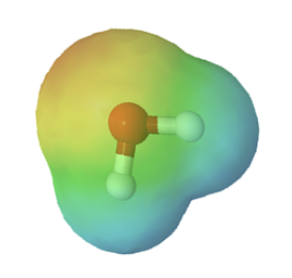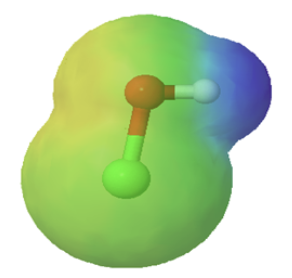water hypochlorous acid

### A "spectrum" of bonds

There is no clear-cut division between covalent and ionic bonds. In a pure non-polar covalent bond, the electrons are held on average exactly half way between the atoms. In a polar bond, the electrons have been dragged slightly towards one end. How far does this dragging have to go before the bond counts as ionic? There is no real answer to that. Sodium chloride is typically considered an ionic solid, but even here the sodium has not completely lost control of its electron. Because of the properties of sodium chloride, however, we tend to count it as if it were purely ionic. Lithium iodide, on the other hand, would be described as being "ionic with some covalent character". In this case, the pair of electrons has not moved entirely over to the iodine end of the bond. Lithium iodide, for example, dissolves in organic solvents like ethanol - not something which ionic substances normally do. Many bonds between metals and non-metal atoms, are considered ionic, however some of these bonds cannot be simply identified as one type of bond. Examples of this are the lithium - carbon bond in methyllithium which is usually considered as polar covalent (somewhat between covalent and ionic) and the potassium - oxygen bond in potassium tert-butoxide which is considered more ionic than covalent.methyllithium potassium tert-butoxide

## Summary

Covalent bonds form when electrons are shared between atoms and are attracted by the nuclei of both atoms. In pure covalent bonds, the electrons are shared equally. In polar covalent bonds, the electrons are shared unequally, as one atom exerts a stronger force of attraction on the electrons than the other. The ability of an atom to attract a pair of electrons in a chemical bond is called its electronegativity. The difference in electronegativity between two atoms determines how polar a bond will be. In a diatomic molecule with two identical atoms, there is no difference in electronegativity, so the bond is nonpolar or pure covalent. When the electronegativity difference is very large, as is the case between metals and nonmetals, the bonding is characterized as ionic.

• No electronegativity difference between two atoms leads to a non-polar covalent bond.
• A small electronegativity difference leads to a polar covalent bond.
• A large electronegativity difference leads to an ionic bond.

HCl+H2OH3O++C

## Exercises

1. Identify the positive and negative ends of each of the bonds shown below.2. Which of the following elements is the more electronegative?

a) Br or C

b) C or H

c) Cl or I

d) C or Li

3. Which of the following molecules would you expect to have the more polarized O-H bond?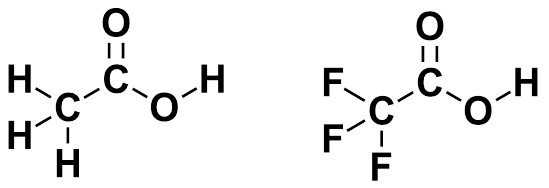4. Predict the direction of polarizing C-O bond in methanol by looking at its electrostatic potential map.## Solutions

1.a) Br

b) C

c) Cl

d) C

3.

The molecule on the right would have the more polorized O-H bond. The presence of the highly electronegative fluorines would draw electrons away by the inductive effect.

4.#### Questions

Q2.1.1

Rank the following from least polar to most polar using knowledge of electronegativity

CH3CH2-Li CH3CH2-K CH3CH2-F CH3CH2-OH

#### Solutions

S2.1.1

(least polar) OH < F < Li < K (most polar)